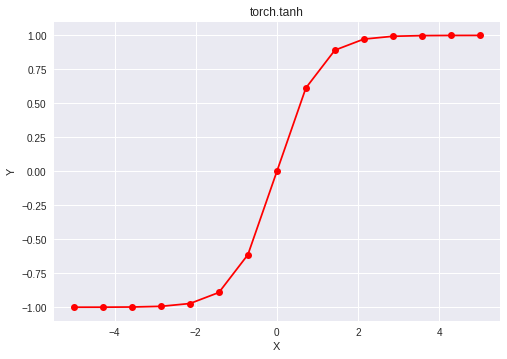# Python | PyTorch tanh() method

PyTorch is an open-source machine learning library developed by Facebook. It is used for deep neural network and natural language processing purposes.

One of the many activation functions is the hyperbolic tangent function (also known as tanh) which is defined as.

The hyperbolic tangent function outputs in the range (-1, 1), thus mapping strongly negative inputs to negative values. Unlike the sigmoid function, only near-zero values are mapped to near-zero outputs, and this solves the “vanishing gradients” problem to some extent. The hyperbolic tangent function is differentiable at every point and its derivative comes out to be. Since the expression involves the tanh function, its value can be reused to make the backward propagation faster.

Despite the lower chances of the network getting “stuck” when compared with the sigmoid function, the hyperbolic tangent function still suffers from “vanishing gradients”. Rectified Linear Unit (ReLU) can be used to overcome this problem.

The function `torch.tanh()` provides support for the hyperbolic tangent function in PyTorch. It expects the input in radian form and the output is in the range [-∞, ∞]. The input type is tensor and if the input contains more than one element, element-wise hyperbolic tangent is computed.

Syntax: torch.tanh(x, out=None)

Parameters:
x: Input tensor
name (optional): Output tensor

Return type: A tensor with the same type as that of x.

Code #1:

 `# Importing the PyTorch library ` `import` `torch ` ` `  `# A constant tensor of size 6 ` `a ``=` `torch.FloatTensor([``1.0``, ``-``0.5``, ``3.4``, ``-``2.1``, ``0.0``, ``-``6.5``]) ` `print``(a) ` ` `  `# Applying the tanh function and ` `# storing the result in 'b' ` `b ``=` `torch.tanh(a) ` `print``(b) `

Output:

``` 1.0000
-0.5000
3.4000
-2.1000
0.0000
-6.5000
[torch.FloatTensor of size 6]

0.7616
-0.4621
0.9978
-0.9705
0.0000
-1.0000
[torch.FloatTensor of size 6]
```

Code #2: Visualization

 `# Importing the PyTorch library ` `import` `torch ` ` `  `# Importing the NumPy library ` `import` `numpy as np ` ` `  `# Importing the matplotlib.pylot function ` `import` `matplotlib.pyplot as plt ` ` `  `# A vector of size 15 with values from -5 to 5 ` `a ``=` `np.linspace(``-``5``, ``5``, ``15``) ` ` `  `# Applying the hyperbolic tangent function and ` `# storing the result in 'b' ` `b ``=` `torch.tanh(torch.FloatTensor(a)) ` ` `  `print``(b) ` ` `  `# Plotting ` `plt.plot(a, b.numpy(), color ``=` `'red'``, marker ``=` `"o"``)  ` `plt.title(``"torch.tanh"``)  ` `plt.xlabel(``"X"``)  ` `plt.ylabel(``"Y"``)  ` ` `  `plt.show() `

Output:

```-0.9999
-0.9996
-0.9984
-0.9934
-0.9728
-0.8914
-0.6134
0.0000
0.6134
0.8914
0.9728
0.9934
0.9984
0.9996
0.9999
[torch.FloatTensor of size 15]
```Attention geek! Strengthen your foundations with the Python Programming Foundation Course and learn the basics.

To begin with, your interview preparations Enhance your Data Structures concepts with the Python DS Course.

My Personal Notes arrow_drop_upCheck out this Author's contributed articles.

If you like GeeksforGeeks and would like to contribute, you can also write an article using contribute.geeksforgeeks.org or mail your article to contribute@geeksforgeeks.org. See your article appearing on the GeeksforGeeks main page and help other Geeks.

Please Improve this article if you find anything incorrect by clicking on the "Improve Article" button below.

Improved By : HaoranShi

Article Tags :

Be the First to upvote.

Please write to us at contribute@geeksforgeeks.org to report any issue with the above content.